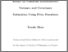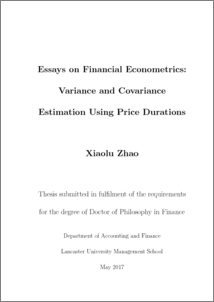# Essays on financial econometrics:variance and covariance estimation using price durations

Zhao, Xiaolu (2017) Essays on financial econometrics:variance and covariance estimation using price durations. PhD thesis, UNSPECIFIED.Preview
PDF (2017ZhaoPhD)
2017ZhaoPhD.pdf - Published Version

## Abstract

Asset variance and covariance are fundamental for financial risk management and many finance applications. With the advent of tick-by-tick high-frequency data, the estimation of univariate variances and multivariate covariance matrices has attracted more attention from econometricians. Many of the proposed high-frequency variance and covariance estimators are based on time-domain measurements. In this thesis, we investigate variance and covariance estimators constructed on the price domain: the price duration based variance and covariance estimators. A price event occurs when the absolute cumulative price change equals or exceeds a pre-specified threshold value. The time taken between two consecutive price events is a price duration. Intuitively, shorter durations are indicative of higher volatility. The duration-based approach provides a new angle to look at the high-frequency data, additionally, the duration based variance and covariance estimators are shown to be more efficient than competing time-domain high-frequency estimators. The information advantage of the duration based approach is demonstrated through two empirical applications, a volatility forecasting exercise and an out-of-sample globalminimum-variance portfolio allocation problem. The duration based estimators are shown to provide both better forecasting performance and better portfolio allocation results. The paper in Chapter 2 is under the first round Revise&Resubmit to the Journal of Business & Economic Statistics. In Chapter 2, we discuss the estimation of univariate variance using price durations. Variance estimation using high-frequency data needs to take into account the effect of market microstructure (MMS) noise, including discrete transaction times, discrete price levels, and bid/ask spreads, as well as price jumps. The price duration estimator has a built-in feature to be robust to large price jumps, while its robustness against the MMS noise is achieved through a careful selection of the threshold value that defines a price event. We discuss the selection of this optimal threshold value through both simulation and empirical evidence. We devise both a non-parametric and a parametric estimator. For the estimation of integrated variance at a daily frequency, the non-parametric duration based variance estimator suffices, while the parametric estimator additionally provides us with an instantaneous variance estimator. As an empirical application to 20 DJIA stocks, we compare the volatility forecasting performance of three classes of volatility estimators, including the realized volatility, the option implied volatility, and the price duration based volatility estimators, on one-day, one-week, and one-month horizons. Forecasting comparisons among individual estimators, as well as in a combination setup, are considered. The duration based estimators, especially the parametric price duration volatility estimator, are found to provide more accurate out-of-sample forecasts. In Chapter 3, we introduce a covariance matrix estimator using price durations. In the multivariate setting, there is the additional issue of nonsynchronous trade arrival times when estimating a high-dimensional variance-covariance matrix using tick-by-tick transaction data. Through simulation, we assess the effects of the lasttick time-synchronization method and MMS noise on the duration based covariance estimator, and compare its accuracy and efficiency with other candidate covariance estimators. Since the covariance matrix is estimated on a pairwise basis, it is not guaranteed to be positive semi-definite (psd). To reduce the number of negative eigenvalues produced by a non-psd matrix, we devise an averaging estimator which is the average of a wide range of duration based covariance matrix estimators. This estimator is applied to a portfolio of 19 DJIA stocks on an out-of-sample global minimum variance portfolio allocation problem where the objective is to minimize the one-day ahead portfolio variance. A simple shrinkage technique is used to improve non-psd and ill-conditioned matrices. The price duration covariance matrix estimator is shown to provide a comparably low portfolio variance while yielding considerably lower portfolio turnover rates than previous estimators.

Item Type:
Thesis (PhD)
Departments:
ID Code:
89003
Deposited By:
Deposited On:
29 Nov 2017 12:46
Refereed?:
No
Published?:
Published